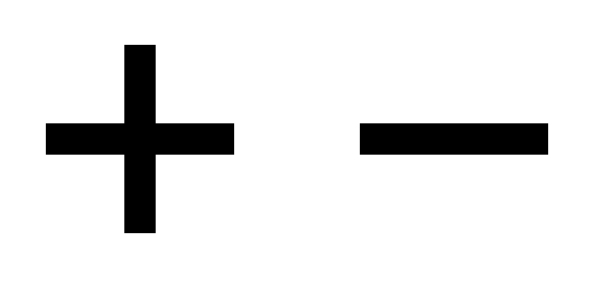20 Questions | Total Attempts: 54Settings• 1.
Choose the correct answer.7 - 4 = 3, so 4 + 3 = ?
• A.

1

• B.

3

• C.

4

• D.

7

• 2.
Choose the correct answer.2 + 8 = 10, so 10 - 2 = ?
• A.

2

• B.

8

• C.

10

• D.

12

• 3.
Choose the correct answer.7 + 4 = 11, so 11 - ? = 7
• A.

4

• B.

10

• C.

11

• D.

18

• 4.
Choose the correct answer.What fact is part of the fact family 5, 9, and 14?
• A.

9 - 4 = 5

• B.

14 + 5 = 19

• C.

9 - 5 = 4

• D.

5 + 9 = 14

• 5.
Find the missing addend.6 + ? = 12
• A.

6

• B.

7

• C.

12

• D.

16

• 6.
Find the missing addend.? + 4 = 13
• A.

3

• B.

9

• C.

10

• D.

15

• 7.
Find the missing addend.8 + ? = 11
• A.

1

• B.

3

• C.

4

• D.

7

• 8.
Find the missing addend.I am a number less than 50.  My tens digit is 2 more than my ones digit.  My ones digit is 1.  What number am I?
• A.

13

• B.

21

• C.

31

• D.

32

• 9.
Use the correct property to solve.8 + 0 = ?
• A.

0

• B.

1

• C.

8

• D.

16

• 10.
Use the correct property to solve.( 3 + 8 ) + 5 = ?
• A.

( 7 + 3 ) + 5

• B.

( 5 + 3 ) + 5

• C.

5 + ( 8 + 5 )

• D.

3 + ( 8 + 5 )

• 11.
Use the correct property to solve.2 + 5 = 5 + ?
• A.

0

• B.

1

• C.

2

• D.

7

• 12.
Find the sum.    27+  36______
• A.

63

• B.

73

• C.

78

• D.

513

• 13.
Find the sum.   77+ 39______
• A.

1,016

• B.

116

• C.

106

• D.

38

• 14.
Find the sum.   95+ 56_____
• A.

39

• B.

141

• C.

151

• D.

1,411

• 15.
Find the sum.Beau has 32 stickers.  His sister has 26.  How many stickers do they have altogether?
• A.

6

• B.

14

• C.

56

• D.

58

• 16.
Find the difference.   48-  19_______
• A.

21

• B.

29

• C.

31

• D.

67

• 17.
Find the difference.   83-  56________
• A.

129

• B.

37

• C.

30

• D.

27

• 18.
Find the difference.There were 64 monkeys at the zoo.  Susan saw 33 monkeys climbing in the trees.  The rest of the monkeys were eating.  How many monkeys were eating?
• A.

21

• B.

31

• C.

37

• D.

97

• 19.
Choose the operation to solve.  Then solve.Jamal collected 48 stamps.  Peter collected 39 stamps.  How many more stamps did Jamal collect than Peter?
• A.

• B.

• C.

Subtraction, 19

• D.

Subbtraction, 9

• 20.
Choose the operation to solve.  Then solve.There were 28 singers in the school chorus last year.  There are 15 more singers in the chorus this year.  How many singers are in the chorus this year?
• A.

• B.Back to top